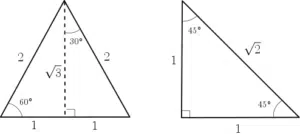# Trigonometric Identities

Trigonometric Identities are identities in mathematics that involve trigonometric functions such as $\sin(x)$, $\cos(x)$ and $\tan(x)$. Identities, as opposed to equations, are statements where the left hand side is equivalent to the right hand side.  We use a $\equiv$ symbol, which means ‘equivalent’, instead of the usual ‘equals’ sign. We solve equations to find values of $x$, for instance. We don’t solve an identity, however, as no additional information has been given. But they can be used to solve equations –  see Trigonometric Equations. It follows that Trigonometric Identities are simply equivalent trigonometric expressions.

## Basic Trigonometric Identities

Exam questions may require you to have memorised some or all of the following basic trigonometric identities and common values:

### Fundamental Identities

$\frac{\sin(\theta)}{\cos(\theta)}\equiv\tan(\theta)$
$\cos^2(\theta)+\sin^2(\theta)\equiv 1$.

$\begin{array}{l}\sin(-\theta)\equiv-\sin(\theta)\\ \cos(-\theta)\equiv\cos(\theta)\\ \sin(90^\circ-\theta)\equiv\cos(\theta)\\ \cos(90^\circ-\theta)\equiv\sin(\theta)\\ \sin(180^\circ-\theta)\equiv\sin(\theta)\\ \cos(180^\circ-\theta)\equiv-\cos(\theta)\\\end{array}$

### Trigonometric Ratios

$\begin{array}{c}\sin(30^\circ)=\frac{1}{2}\\\cos(30^\circ)=\frac{\sqrt{3}}{2}\\\tan(30^\circ)=\frac{\sqrt{3}}{3}\\\\\sin(45^\circ)=\frac{\sqrt{2}}{2}\\\cos(45^\circ)=\frac{\sqrt{2}}{2}\\\tan(45^\circ)=1\\\\\sin(60^\circ)=\frac{\sqrt{3}}{2}\\\cos(60^\circ)=\frac{1}{2}\\\tan(60^\circ)=\sqrt{3}\end{array}$

## Where do these Trigonometric Identities come from?

### Fundamental Identities

Firstly, the identity $\tan(\theta)\equiv\frac{\sin(\theta)}{\cos (\theta)}$ can be seen using SOHCAHTOA. Recall that

$\sin(\theta)=\frac{OPP}{HYP}$ and  $\cos(\theta)=\frac{ADH}{HYP}$.

It follows that $\tan(\theta)=\frac{OPP}{ADJ}=\frac{OPP}{HYP}\div \frac{ADJ}{HYP}=\frac{\sin(\theta)}{\cos(\theta)}$.

### Trigonometric Ratios

The Trigonometric Ratios seen above can be found without using a calculator. We can find them from two ‘special triangles’:## More Trigonometric Identities

In addition to the above, you may be require to master the following identities. These identities are more complicated than the ones seen above and so may feature later on in your course.

### Double Angle Identities

$\begin{array}{l}\sin(2\theta)\equiv2\sin(\theta)\cos(\theta)\\\cos(2\theta)\equiv\cos(\theta)^2-\sin^2(\theta)\\\cos(2\theta)\equiv2\cos^2(\theta)-1\\\cos(2\theta)\equiv1-2\sin^2(\theta)\end{array}$

### Compound Angle Indentities

$\begin{array}{l}\sin(\alpha\pm\beta)\equiv\sin(\alpha)\cos(\beta)\pm\cos(\alpha)\sin(\beta)\\\cos(\alpha\pm\beta)\equiv\sin(\alpha)\sin(\beta)\mp\cos(\alpha)\cos(\beta)\\\tan(\alpha\pm\beta)\equiv\frac{\tan(\alpha)\pm\tan(\beta)}{1\mp\tan(\alpha)\tan(\beta)}\end{array}$

For more trigonometric identities visit Wikipedia Trig. Identities.

## Examples on Trigonometric Identities

Simplify

$\frac{\sqrt{1-\cos^2(x)}}{\cos(x)}$

Show that $\frac{\sin^4(\theta)-\cos^4(\theta)}{\cos^2(\theta)}\equiv\tan^2(\theta)-1$.

Given that $\theta$ is obtuse and $\cos(\theta)=-\frac{2}{5}$, find the value of $\tan(\theta)$.

Given that $x=2\cos(\alpha)$ and $y=3\sin(\alpha)$, find the value of $9x^2+4y^2$.